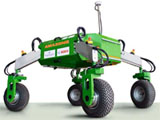## Biological Systems Engineering## Biological Systems Engineering: Papers and Publications

4-1980

#### Citation

General Agriculture, B-5 Public Policy, Issued April 1980

#### Abstract

The metric and U.S. customary systems of measure differ in terminology for fluid volume, mass, temperature, pressure, power and energy. The relationships between the metric and U.S. customary system for these units are described and illustrated graphically in the following text.

Fluid Volume- Fluid volumes are usually measured in milliliters or liters. Small quantities of liquid products are expressed as milliliters or as cubic centimeters. Gasoline, milk and other similar sized volumes are measured in liters.

Mass - Mass is the amount of matter in an object and is normally referred to as weight. The common metric units of mass are milligram, gram, kilogram and tonne (1,000 kilograms). Medicine, vitamins and other small objects are measured in milligrams. Several food products are measured in grams. Heavier objects, including larger food quantities and humans, have masses expressed in kilograms. A truck load of material and other large quantities of matter are measured in tonnes.

Mass and volume are related in the metric system. A milliliter of water at 4°C (39.2°F) and at sea level is equal to a cubic centimeter of water and has a mass of one gram. A liter of water equal to 1,000 cubic centimeters has a mass of one kilogram, and a cubic meter of water has a mass of one tonne.

Temperature - Temperature in the metric system is measured in degrees Celsius (formerly called centigrade). Water freezes at 0°C (32°F) and boils at 100°C (212°F). Normal body temperature is 37°C (98.6 °F). A temperature of -5 °F is equal to -20.5 °C.

Pressure - Pressure, which is force per unit area, is measured in pascals. One pascal is a very small pressure, so kilopascal is the preferred unit for most commercial and industrial applications. Standard atmospheric pressure of 14.7 pounds per square inch is equal to 101 kilopascals.

Power- The unit of power in the metric system is the watt. Conventionally, we use entirely different units for power in mechanical, thermal and electrical systems. In the metric system, however, all power is measured in watts. A tractor rated at 100 horsepower in the U.S. customary system will have a rating of 74.6 kilowatts in the metric system.

Energy, Quantity of Heat - All forms of energy in the metric system are ex-pressed as joules. The nutrition calorie, which equals one kilocalorie, is replaced by the kilojoule, with one kilocalorie being equal to approximately four kilojoules. Larger heat quantities such as furnaces and air conditioners are measured in megajoules (1,000 kilo joules). Kilowatt-hour, a commonly used measure of energy, is replaced with the megajoule. One kilowatt-hour equals 3.6 megajoules.

COinS